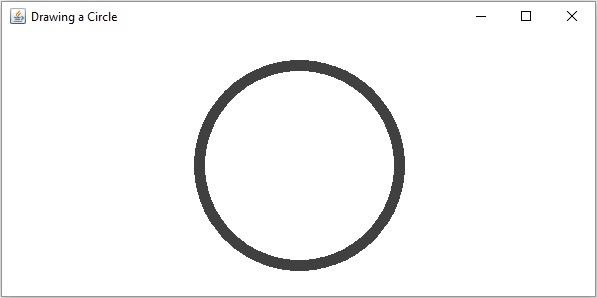# How to draw a circle in OpenCV using Java?

The org.opencv.imgproc package of Java OpenCV library contains a class named Imgproc.

To draw a circle you need to invoke the circle() method of this class. This method accepts the following parameters −

• A Mat object representing the image on which the circle is to be drawn.

• A Point object representing the center of the circle.

• An integer variable representing the radius of the circle.

• A Scalar object representing the color of the circle(BGR).

• An integer representing the thickness of the circle(default 1).

## Example

import org.opencv.core.Core;
import org.opencv.core.Mat;
import org.opencv.core.Point;
import org.opencv.core.Scalar;
import org.opencv.highgui.HighGui;
import org.opencv.imgcodecs.Imgcodecs;
import org.opencv.imgproc.Imgproc;
public class DrawingCircle {
public static void main(String args[]) {
//Reading the source image in to a Mat object
String file ="D:\images\blank.jpg";
//Drawing a Circle
Point center = new Point(300, 200);
Scalar color = new Scalar(64, 64, 64);
int thickness = 10;
Imgproc.circle (src, center, radius, color, thickness);
//Saving and displaying the image
Imgcodecs.imwrite("circle.jpg", src);
HighGui.imshow("Drawing a Circle", src);
HighGui.waitKey();
}
}

## Output

On executing, the above program generates the following window −